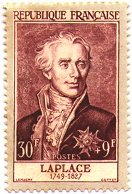Search IntMath
Close

# The Laplace TransformationPierre-Simon Laplace (1749-1827)

Laplace was a French mathematician, astronomer, and physicist who applied the Newtonian theory of gravitation to the solar system (an important problem of his day). He played a leading role in the development of the metric system.

The Laplace Transform is widely used in engineering applications (mechanical and electronic), especially where the driving force is discontinuous. It is also used in process control.

## What Does the Laplace Transform Do?

The main idea behind the Laplace Transformation is that we can solve an equation (or system of equations) containing differential and integral terms by transforming the equation in "t-space" to one in "s-space". This makes the problem much easier to solve. The kinds of problems where the Laplace Transform is invaluable occur in electronics. You can take a sneak preview in the Applications of Laplace section.

If needed we can find the inverse Laplace transform, which gives us the solution back in "t-space".

## What's in this Chapter?

1a. The Unit Step Function - Definition (otherwise known as Heaviside function)

1b. The Unit Step Function - Products (how to "turn on" or "turn off" signals at different times)

2. Laplace Transform Definition (as an infinite integral)

Table of Laplace Transformations (an easier way to find Laplace Transforms)

3. Properties of Laplace Transform (with worked examples)

4. Transform of Unit Step Functions

5. Transform of Periodic Functions (like sine and cosine)

We begin the chapter by introducing the topic of Unit Step Functions »

## Problem SolverThis tool combines the power of mathematical computation engine that excels at solving mathematical formulas with the power of GPT large language models to parse and generate natural language. This creates math problem solver thats more accurate than ChatGPT, more flexible than a calculator, and faster answers than a human tutor. Learn More.